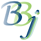# Object Variables

## Description

Object variables are similar to string, numeric and integer variables; they act as holders for data. An object variable is a universal holder; it can contain any kind of data. Object variables are distinguished from other variable types by an exclamation mark at the end of the name. Some examples of object variables are:

 X! = "The quick brown fox" Y! = 12.95 Z! = new java.util.HashMap()

In this example, X! is an object variable that contains a string value. It can be used almost everywhere a string variable can be used. For example:

 PRINT X!; REM ' this is equivalent to PRINT X!.toString() PRINT LEN(X!); REM ' equivalent to PRINT X!.toString().length() PRINT POS("brown"=X!)

To avoid conflict between BBj template and substring syntax versus object syntax, object variables cannot be dimensioned with string templates, and they do not support BBx substring notation.

All of the following examples are syntax errors:

 DIM X!:"FIELD:C(1*)"; REM ' can't dim object variable with a template PRINT X!(6,6); REM ' object variables don't support substring notation LET X!(11,6)="green"; REM ' substring notation isn't supported

Object variables cannot be used in INPUT or DREAD statements.

Y! is an object variable that contains a numeric value. In can be used almost everywhere a numeric variable can be used. For example:

 PRINT Y!:"###.00"; REM ' prints " 12.95" PRINT Y!*2; REM ' prints 25.9 PRINT -Y!; REM ' prints –12.95

An object variable is treated as a number if it was created from a numeric expression, or from a Java call that returned a Java primitive type (boolean, byte, char, short, int, long, float or double). A boolean value of false becomes zero and a boolean value of true becomes one.

See Calling Java From BBj for more details.

Z! is a Java object. There are several ways to create a Java object. The first method is to use the 'new' operator. This works the same way in BBj as in Java:

 map! = new java.util.HashMap() int! = new Integer(123) array! = new int; rem ' Java array, BBj 18.0 and higher

The BBjAPI() function is an entry point to many object-oriented features of the BBj language. For example:

 BBJAPI! = bbjapi() SYSGUI! = BBJAPI!.getSysGui() CONTROL! = SYSGUI!.getWindow(101).getControl(12) CONTROL!.setLocation(3,12)

Public attributes of an object can be accessed using dot notation. For example:

 DIM! = new java.awt.Dimension(10,20) X! = DIM!.height

Public methods of an object can be accessed using dot notation. For example:

 A! = new java.util.ArrayList() X! = "Hello, World!"A!.add(X!)X = A!.size()

Static attributes and methods of any Java class can also be accessed. For example:

 System.out.println("message sent to stdout") PI = Math.PI Locale! = java.util.Locale.getDefault()

The NULL() Function returns a Java null value. This can be used to compare a returned value to null. For example:

 0010 h! = new java.util.HashMap() 0020 h!.put("NM","New Mexico") 0030 IF h!.get("NM")<>NULL() THEN PRINT "NM found" 0040 IF h!.get("AZ")=NULL() THEN PRINT "AZ not found" >run NM found AZ not found

Uninitialized object variables are equal to null:

 >START READY >PRINT X! null >